ZHCSJE3C February   2019  – August 2021

PRODUCTION DATA

1. 特性
2. 应用
3. 说明
4. Revision History
5. Pin Configuration and Functions
6. Specifications
7. Detailed Description
1. 7.1 Overview
2. 7.2 Functional Block Diagram
3. 7.3 Feature Description
4. 7.4 Device Functional Modes
8. Application and Implementation
1. 8.1 Application Information
2. 8.2 Typical Application
1. 8.2.1 Microamp Current Measurement
9. Power Supply Recommendations
10. 10Layout
11. 11Device and Documentation Support
12. 12Mechanical, Packaging, and Orderable Information

• YFD|6

## 6.6 Typical Characteristics

at TA = 25 °C, VS = 1.8 V, VIN+ = 12 V, VENABLE = VS, VREF = GND and all gain options (unless otherwise noted)Figure 6-1 Input Offset Voltage Production DistributionFigure 6-3 Common-Mode Rejection Production DistributionA1 devices
Figure 6-5 Gain Error Production Distribution (INA191)A2, A3, A4, A5 devices
Figure 6-7 Gain Error Production DistributionVS = 5 V
Figure 6-9 Gain vs. Frequency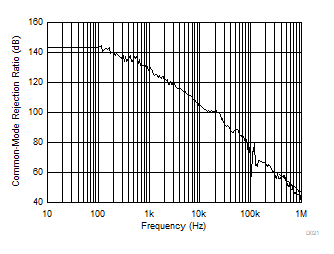Figure 6-11 Common-Mode Rejection Ratio vs. FrequencyVS = 5.0 V
Figure 6-13 Output Voltage Swing vs. Output CurrentVENABLE = 0 V, VSENSE = 0 V
Figure 6-15 Input Bias Current vs. Common-Mode Voltage (Shutdown)VENABLE = VS
Figure 6-17 Quiescent Current vs. Temperature (INA191)VENABLE1 = VENABLE2 = VS, VREF = VS /2
Figure 6-19 Quiescent Current vs. Temperature (INA2191 )VENABLE1 = VENABLE2 = 0 V, VREF = VS /2
Figure 6-21 Quiescent Current vs. Temperature (INA2191 Disabled)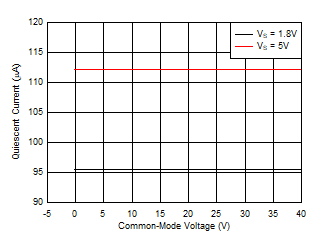VREF = VS /2
Figure 6-23 Quiescent Current vs. Common-Mode Voltage (INA2191)Figure 6-25 0.1-Hz to 10-Hz Input-Referred Voltage Noise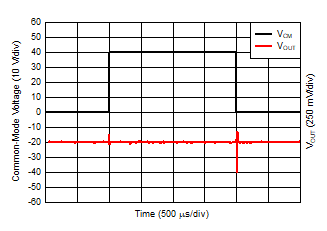Figure 6-27 Common-Mode Voltage Transient ResponseVS = 5.0 V
Figure 6-29 Noninverting Differential Input Overload Recovery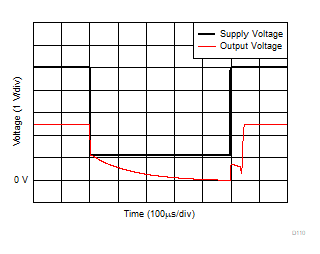VS = 5.0 V, A3 device
Figure 6-31 Brownout Recovery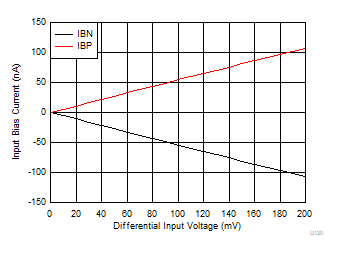VS = 5.0 V, A1 device
Figure 6-33 IB+ and IB– vs. Differential Input Voltage (INA191)VS = 5.0 V, VREF = VS /2, A1 device
Figure 6-35 IB+ and IB– vs. Differential Input Voltage (INA2191)VS = 5.0 V, VENABLE = 0 V, A1, A2, A3 devices
Figure 6-37 Output Leakage vs. Output VoltageVS = 5.0 V, VENABLE = 0 V, VREF = 2.5 V, A1, A2, A3 devices
Figure 6-39 Output Leakage vs. Output Voltage (INA2191)VS = 5.0 V, VCM = 0 V
Figure 6-41 Output Impedance vs. FrequencyFigure 6-2 Offset Voltage vs. TemperatureFigure 6-4 Common-Mode Rejection Ratio vs. TemperatureA1 Devices
Figure 6-6 Gain Error Production Distribution (INA2191)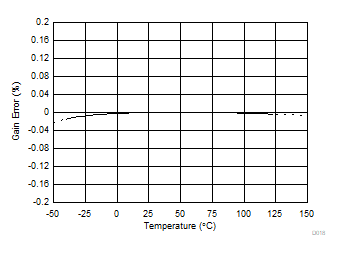Figure 6-8 Gain Error vs. TemperatureVS = 5 V
Figure 6-10 Power-Supply Rejection Ratio vs. FrequencyVS = 1.8 V
Figure 6-12 Output Voltage Swing vs. Output CurrentVS = 5.0 V, VSENSE = 0 V
Figure 6-14 Input Bias Current vs. Common-Mode Voltage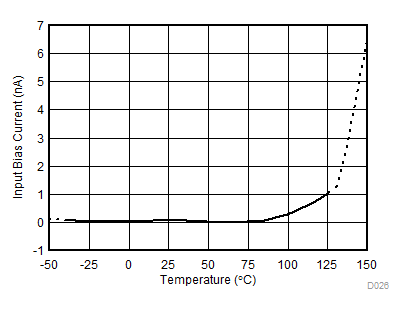VSENSE = 0 V
Figure 6-16 Input Bias Current vs. TemperatureSingle channel enabled, VREF = VS /2
Figure 6-18 Quiescent Current vs. Temperature (INA2191 )VENABLE = 0 V
Figure 6-20 Quiescent Current vs. Temperature (INA191 Disabled)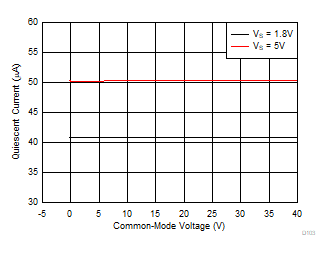Figure 6-22 Quiescent Current vs. Common-Mode Voltage (INA191)Figure 6-24 Input-Referred Voltage Noise vs. Frequency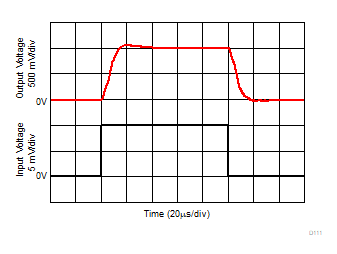VS = 5.0 V, 10-mVPP input step
Figure 6-26 Step ResponseFigure 6-28 Inverting Differential Input Overload RecoveryVS = 5.0 V, A2 device
Figure 6-30 Start-Up Response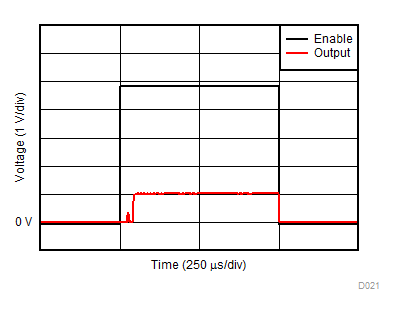VS = 5.0 V, A3 device
Figure 6-32 Enable and Disable ResponseVS = 5.0 V, A2, A3, A4, A5 devices
Figure 6-34 IB+ and IB– vs. Differential Input Voltage (INA191)

 VS = 5.0 V, VREF = VS /2, A2, A3, A4, A5 devices
Figure 6-36 IB+ and IB– vs. Differential Input Voltage (INA2191)VS = 5.0 V, VENABLE = 0 V, A4, A5 devices
Figure 6-38 Output Leakage vs. Output VoltageVS = 5.0 V, VENABLE = 0 V, VREF = 2.5 V, A4, A5 devices
Figure 6-40 Output Leakage vs. Output Voltage (INA2191)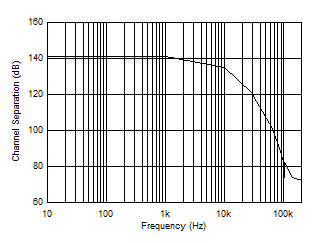VS = 5.0 V, VCM = 0 V, VREF = VS /2
Figure 6-42 Channel Separation vs. Frequency (INA2191)